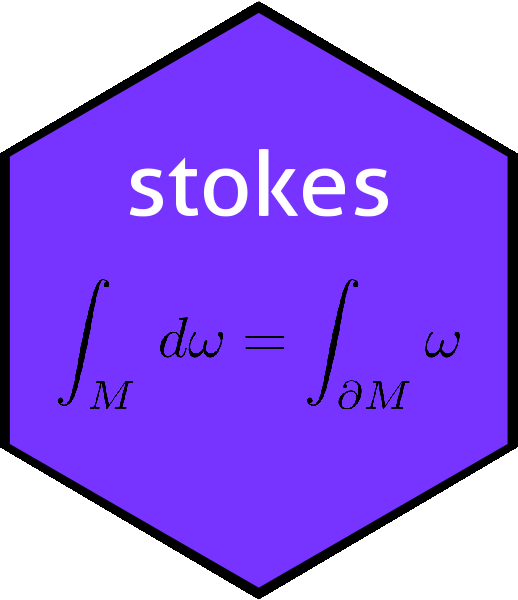# Function volume() in the Stokes packagevolume
## function (n)
## {
##     as.kform(seq_len(n))
## }
## <bytecode: 0x7fc3a58dc838>
## <environment: namespace:stokes>

Spivak, in a memorable passage, states (p83):

The volume element

The fact that $$\operatorname{dim}\Lambda^n\left(\mathbb{R}^n\right)=1$$ is probably not new to you, since $$\operatorname{det}$$ is often defined as the unique element $$\omega\in\Lambda^n\left(\mathbb{R}^n\right)$$ such that $$\omega\left(e_1,\ldots,e_n\right)=1$$. For a general vector space $$V$$ there is no extra criterion of this sort to distinguish a particular $$\omega\in\Lambda^n\left(\mathbb{R}^n\right)$$. Suppose, however, that an inner product $$T$$ for $$V$$ is given. If $$v_1,\ldots,v_n$$ and $$w_1,\ldots, w_n$$ are two bases which are orthonormal with respect to $$T$$, and the matrix $$A=\left(a_{ij}\right)$$ is defined by $$w_i=\sum_{j=1}^n a_{ij}v_j$$, then

$\delta_{ij}=T\left(w_i,w_j\right)= \sum_{k,l=1}^n a_{ik}a_{jl}T\left(v_k,v_l\right)= \sum_{k=1}^n a_{ik}a_{jk}.$

In other words, if $$A^T$$ denotes the transpose of the matrix $$A$$, then we have $$A\cdot A^T=I$$, so $$\operatorname{det}A=\pm 1$$. It follows from Theorem 4-6 [see vignette det.Rmd] that if $$\omega\in\Lambda^n(V)$$ satisfies $$\omega\left(v_1,\ldots,v_n\right)=\pm 1$$, then $$\omega\left(w_1,\ldots,w_n\right)=\pm 1$$. If an orientation $$\mu$$ for $$V$$ has also been given, it follows that there is a unique $$\omega\in\Lambda^n(V)$$ such that $$\omega\left(v_1,\ldots,v_n\right)=1$$ whenever $$v_1,\ldots,v_n$$ is an orthornormal basis such that $$\left[v_1,\ldots,v_n\right]=\mu$$. This unique $$\omega$$ is called the volume element of $$V$$, determined by the inner product $$T$$ and orientation $$\mu$$. Note that $$\operatorname{det}$$ is the volume element of $$\mathbb{R}^n$$ determined by the usual inner product and usual orientation, and that $$\left|\operatorname{det}\left(v_1,\ldots,v_n\right)\right|$$ is the volume of the parallelipiped spanned by the line segments from $$0$$ to each of $$v_1,\ldots,v_n$$.

- Michael Spivak, 1969 (Calculus on Manifolds, Perseus books). Page 89

In the stokes package, function volume(n) returns the volume element on the usual basis, that is, $$\omega\left(e_1,\ldots,e_n\right)$$. We will take $$n=7$$ as an example:

(V <- volume(7))
## An alternating linear map from V^7 to R with V=R^7:
##                    val
##  1 2 3 4 5 6 7  =    1

We can verify Spivak’s reasoning as follows:

f <- as.function(V)
f(diag(7))
##  1

Above, we see that $$\omega\left(e_1,\ldots,e_n\right)=1$$. To verify that $$V\left(v_1,\ldots,v_n\right)=\operatorname{det}(A)$$, where $$A_{ij}=\left(v_i\right)_j$$:

A <- matrix(rnorm(49),7,7)
LHS <- f(A)
RHS <- det(A)
c(LHS=LHS,RHS=RHS,diff=LHS-RHS)
##      LHS      RHS     diff
## 1.770074 1.770074 0.000000

Now we create $$w_1,\ldots,w_n$$, another orthonormal set. We may verify by generating a random orthogonal matrix and permutiting its rows:

M1 <- qr.Q(qr(matrix(rnorm(49),7,7)))  # M1: a random orthogonal matrix
M2 <- M1[c(2,1,3,4,5,6,7),]            # M2: (odd) permutation of rows of M1
c(f(M1),f(M2))
##   1 -1

Above we see that the volume element of M1 and M2 are $$\pm1$$ to within numerical precision.